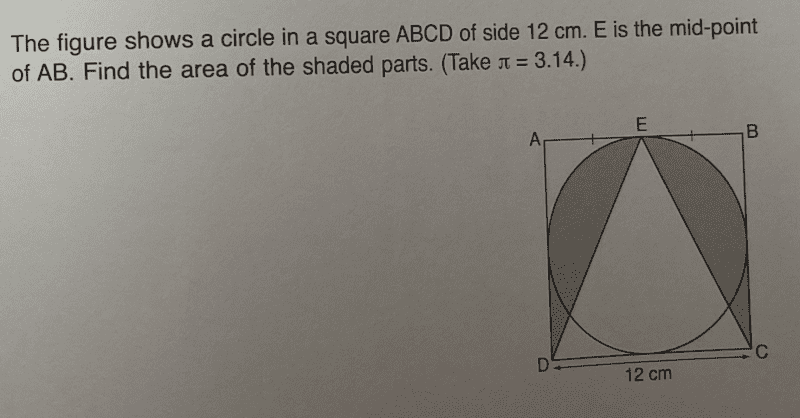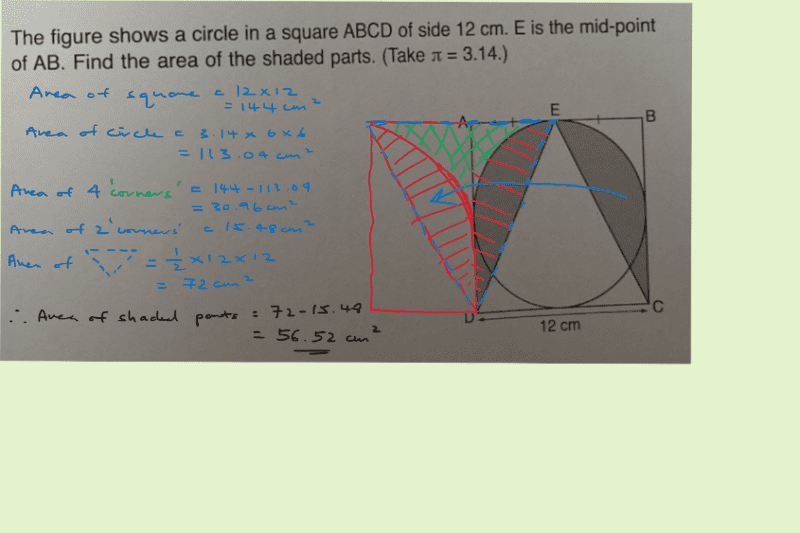# QuestionLet M be the midpoint between A and D.

Area of quadrant AEM = (1/4) pi r² = (1/4) (3.14) (6)² = 28.26 cm²

Area of square AEM = 6×6 cm² = 36 cm²

so, area of white area AEM = 36 – 28.26 = 7.74 cm²

Area of triangle EDC = 0.5  x 12 x 12 = 72 cm²

Area of whole square ABCD = 12 x 12 = 144 cm²

So area of shaded areas = 144 – 72 – 2(7.74) cm² =  56.52 cm² #

0 Replies 2 Likes ✔Accepted Answer0 Replies 1 Like